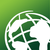# Rental dwellings average calculation methodology

107
0
01-20-2021 10:01 AM
Labels (1)by
New Contributor
I came across a possible error in ESRI's calculations. We believe the rental dwelling and maintenance repair material and other rental dwelling statistics calculate averages based on total housing units in the area, not just rental units. If this is accurate, all nonrental housing units severely skew all averages. I attached the file showing costs and 2020 renter-occupied household units.

You'll notice the average in column C is extremely low. If you multiply the average by the number of 2020  renter-occupied household units, you get nowhere near the annual total spent. However, in row 3, if you divide the total spent (B3) by the average (C3), you get 353, the same as if you divide the number of 2020 renter-occupied household units (D3)  by the percentage of renter occupied household units (D4). These numbers are equal along all rows of block groups. I included these calculations in the attached sheet. I believe this means the average in column C is calculated based on the block group's total housing units. In my opinion, the average should be calculated using the values of 2020 renter-occupied household units. I hypothesize this severely skews the average as non-rented households would not have any value associated with rental maintenance. I think this may be true across all rental dwelling statistics.

Are we misinterpreting the average? What's the best way to upload my own averages and generate those maps?
0 Replies